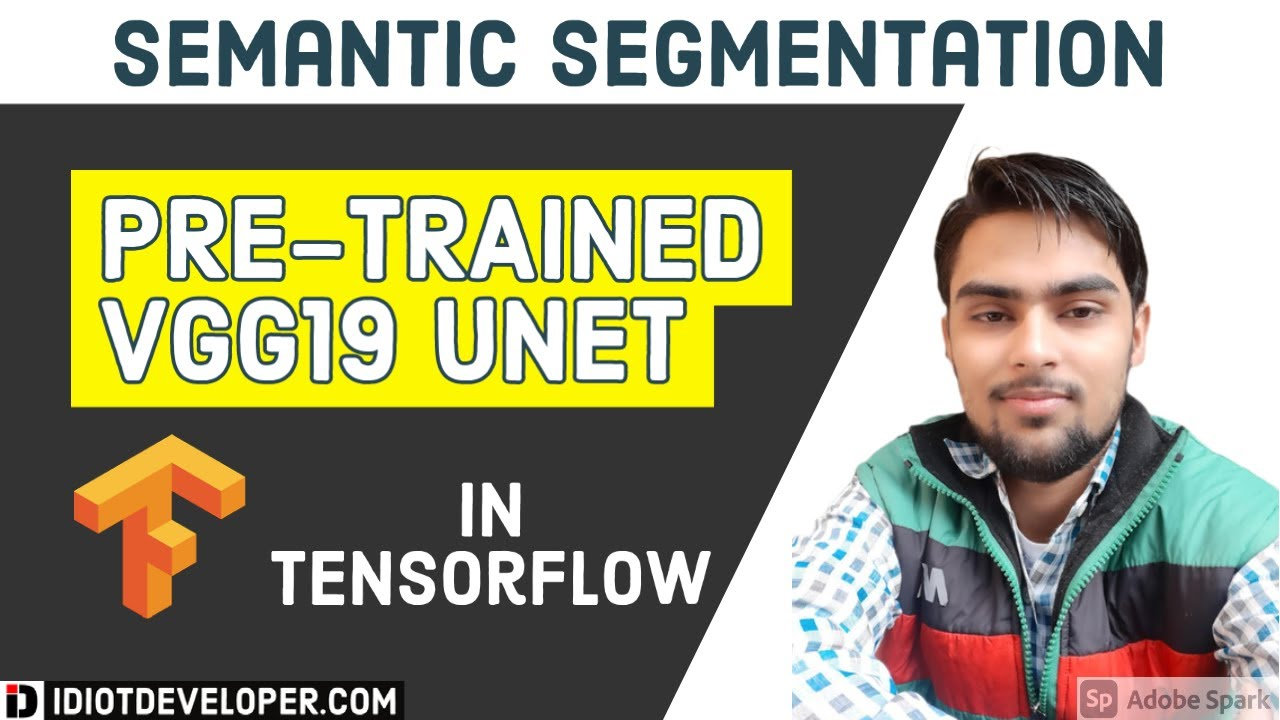# Pretrained VGG19 UNET in TensorFlow using Keras | Semantic Segmentation | Deep LearningIn this video, we are going to implement UNET in TensorFlow using Keras API. Here we are going to replace the encoder part of the UNET with a pre-trained VGG19 architecture.

In this video, we are going to implement UNET in TensorFlow using Keras API. Here we are going to replace the encoder part of the UNET with a pre-trained VGG19 architecture.

## Keras vs. Tensorflow - Difference Between Tensorflow and Keras

Keras vs Tensorflow - Learn the differences between Keras and Tensorflow on basis of Ease to use, Fast development,Functionality,flexibility,Performance etc

## TensorFlow And Keras Tutorial | Deep Learning With TensorFlow & Keras | Deep Learning

This video on TensorFlow and Keras tutorial will help you understand Deep Learning frameworks, what is TensorFlow, TensorFlow features and applications, how TensorFlow works, TensorFlow 1.0 vs TensorFlow 2.0, TensorFlow architecture with a demo. Then we will move into understanding what is Keras, models offered in Keras, what are neural networks and they work.

## Pytorch vs Tensorflow vs Keras | Deep Learning Tutorial (Tensorflow, Keras & Python)

We will go over what is the difference between pytorch, tensorflow and keras in this video. Pytorch and Tensorflow are two most popular deep learning frameworks. Pytorch is by facebook and Tensorflow is by Google. Keras is not a full fledge deep learning framework, it is just a wrapper around Tensorflow that provides some convenient APIs.

## Keras Tutorial - Ultimate Guide to Deep Learning - DataFlair

Keras Tutorial - Learn Keras Introduction, installation, Keras Features, Applications of Keras, Keras Layers, Keras models and keras visualize training.

## Keras Tutorial For Beginners | What is Keras | Keras Sequential Model | Keras Training

🔵 Intellipaat Artificial Intelligence Master's Course: https://intellipaat.com/artificial-intelligence-masters-training-course/🔵 In this video on Keras, ...